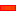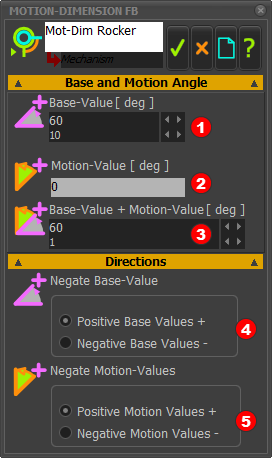﻿ Step 5.3: The Motion-Dimension FB

# Step 5.3: The Motion-Dimension FB

## Motion-Dimension FB

A Motion-Dimension FB identifies a Part to which we can give a motion.

When we add a Motion-Dimension FB to a Part, we call the Part a Motion-Part.

There are two types of Motion-Parts:

 • Rocker  = Part + Pin-Joint + Motion-Dimension FB
 • = Part + Slide- Joint + Motion-Dimension FB

### Key Learnings in this Step.

We add a Motion-Dimension FB to give a Slider and a Rocker.

Two values define the position of the Motion-Part.

Motion-Dimension = Base-Value + Motion-Values

 • Base-Value : the value we can edit  - it is the position of the Part if we do not connect a wire to the input-connector
 • Motion-values : the position [and velocity and acceleration] at the input-connector

####Add Motion-Dimension FBKinematics-FB toolbar > Add Motion-Dimension FBThe Command-Manager has three selection-boxes.Click a Joint [Slide-Joint or Pin-Joint]Click a solved Line or Point [in a Green Part]Click an unsolved Line or Point [in a Blue Part*] Notes:are Lines ifis a Pin-Jointare Points ifis a Slide-Jointa Line or a Point in a kinematically-defined Part. The Parts have a Green Part-Outlinesa Line or a Point in a Free-Part [a Part that is NOT kinematically-defined]. The Parts have Blue Part-Outlines.

####Motion Dimension FB in the graphic-area

 The icon of the FB in the graphic-area is different to the icon in the Kinematic-FB toolbar.The Motion-Dimension FB that identifies a RockerThe Motion-Dimension FB that identifies a Slider

####Motion-Dimension FB and a ROCKER

PREPARATION:Part joined to the Base-PartPart joined to a Rocker

Before you can add the Motion-Dimension FB, you must have, at minimum of:

 1 Two Parts• One Part must be a kinematically-defined Part

and

 • The other Part must be Free-Part
 2 A Pin-Joint3 The Pin-Joint joins Points from Linesin each of the two PartsTwo examples to the left:

 • a Free-part joined to the Base-Part [TOP IMAGE]
 • a Free-Part joined to a Rocker [BOTTOM IMAGE]

SELECT ELEMENTS: Element 1: PIN-JOINTKinematic-FB toolbar > Add Motion-Dimension FB

In the Command-Manager: You have the option to select a Pin-Joint or a Slide-Joint as the first element.

For the Part to become a Rocker, you must select a Pin-Joint.

Rule 1 [Rocker]

 • Element 1: a Pin-Joint

The Pin-Joint joins a kinematically-defined Part to a Free Part

Rule 2 [Rocker]

 • The Pin-Joint must join Points that are at the ends of Lines

SELECT ELEMENTS: Elements 2 & 3: LINESRule 3 [Rocker]

 • Element 2: a Line

This Line radiates from the Pin-Joint [Element 1] and is in the kinematically-defined Part

 • Element 3: a Line

This Line radiates from the Pin-Joint [Element 1] and is in the Free Part

Note:

To complete the command:

 • Left-Click thein the Command-Manager .

The Motion-Dimension FB icon is at the left of the graphic-area, and not a very convenient place.

or

 • GOOD SHORTCUT - Right-Click with your pointer in the graphic-area.

The Motion-Dimension FB icon is near to your mouse-pointer - much more convenient.

RESULT: The Motion-Dimension FB and a Pin-Joint.The Free-Part becomes a Rocker

The Rocker is kinematically-defined, with a Green Part-OutlineIcon for a Rocker in the graphic-area.

To edit the Motion-Dimension FB

 1 Double-click the Motion-Dimension FB- or -

 1 Double-click the Motion-Dimension, or its arrowhead, but not the dimension value itself.

- or -Tutorial 1: Step 1.5

 • Edit a Motion-Dimension FB

Notes:

To move the Motion-Dimension Function-Block:

 • Hover + Drag the Motion-Dimension Function-Block

To move the Motion-Dimension:

 • CTRL + Hover + Drag the arrowhead of the Motion-Dimension.

####Motion-Dimension FB and a SLIDE-JOINT

PREPARATIONBefore you can add the Motion-Dimension FB, you must have, at minimum of:

 1 Two Parts
 • One Part must be a kinematically-defined Part

This example, the kinematically-defined Part is the Base-Part

and

 • The other Part must be Free-Part
 2 A Slide-Joint
 3 The Slide-Joint joins Lines in each of the two Parts

Note:

The arrowhead shows the Positive direction of the Slide-Joint.

SELECT ELEMENTS: Element 1: SLIDE-JOINTRule 1 [Slider]

 • Element 1: A Slide-Jointjoins a kinematically-defined Part to a Free Part

SELECT ELEMENTS: Elements 2&3: POINTSAfter you select a Slide-Joint as Element 1, you must select Points as Elements 2&3.

Rule 2 [Slider]:

 • Element 2: a Point

This Point is at one end of the Line in the kinematically-defined Part

Rule 3 [Slider]:

 • Element 3: a Point

This Point is at one end of the Line in the Free Part.

RESULT: The Motion-Dimension FB applied to a Slide-Joint.The Free-Part becomes a Slider

The Slider is kinematically-defined, with a Green Part-OutlineThe Motion-Dimension FB icon for the Slider in the graphic-area.

To edit the Motion-Dimension FB

 1 Double-click the Motion-Dimension FB- or -

 1 Double-click the Motion-Dimension, or its arrowhead, but not the dimension value itself.

- or -Notes:

To move the Motion-Dimension Function-Block:

 • Hover + Drag the Motion-Dimension Function-Block

To move the Motion-Dimension:

 • CTRL + Hover + Drag the arrowhead of the Motion-Dimension.

####Motion Dimension FB dialog boxMotion Dimension FB

You cannot specify the initial position of the Motion-Part as you add a Motion-Dimension FB.

To edit the initial position of the Motion-Part - we call it the Base-Value - we must open Motion-Dimension FB dialog-box.

To edit the Motion-Dimension FB:

 1 Double-click the Motion-Dimension FB in the graphic-area

The Motion-Dimension FB dialog-box opens.

 2 Edit the:
 o Base-Value- or -

 o Base-Value + Motion ValueIt is best to think of the:

 • Base-Value as the position of the Motion-Part when a wire is not connected to the input-connector.
 • Base-Value + Motion-Value as the position of the Motion-Part when a wire is connected to the input-connector.

Directions  separator, there are options to:

 • Edit the positive direction of the Base-Value
 • Edit the positive direction of the Motion-Values.

####Use a Graph FB to display the values at the output-connector of a Motion-Dimension FB

 STEP 1: Add TWO Linear-Motion Function-Blocks to the graphic-area.
 STEP 2: Add a Graph FB to the Mechanism graphic-area
 STEP 3: Connect the Linear-Motion FB to the Graph FB X-axis (the bottom connector).
 STEP 4: Edit the Base-Part and add a Line - See Step 1.3
 STEP 5: Add a Part (Step 1.2) and a Pin-Joint (Step 1.4) to join one end of the Part to the end of a Line in the Base-Part.
 STEP 6: Add a Motion-Dimension FB which identifies Added-Part as the Part we will specify an angle relative to the Line in the Base-Part.
 STEP 7: Connect the other Linear-Motion FB to the Motion-Dimension FB, and from the Motion-Dimension FB to the Graph Y-axis
 STEP 8: Double-click the Linear-Motion FB: Edit the phase to 30
 STEP 9: Double-click the Motion-Dimension FB, Edit the Base-Value of the Motion-Dimension to 110
 STEP 10: Double-click the Graph FB to show the plot of the variables.The image shows the elements in the graphic-area after Steps 1-10.

The 'top' Linear-Motion FBadds 30 to the MMA.

We have connected a wire from the Linear-Motion FB directly to Motion-Dimension FBWe have connected a wire from the Motion-Dimension FB directly to the Y1 input of the Graph FB.

The Motion-Dimension dialog-boxand the Graph FBare open.

The Base-Value of the Motion-Dimension is 110ºin the Motion-Dimension dialog-box.

The Master-Machine-Angle (MMA) is 120º

Thus, the output from the Linear-Motion FB is 120º + 30º = 150º.

This means the input to the Motion-Dimension FB = 150º.

The Base-Value for the Motion-Dimension is 110º.

Thus, the Rocker angle is at 110º + 150º = 260º.

The output from the Motion-Dimension FB is also the input to the Graph FB.

When you open the Graph FB, the red cursor is at the MMA.

If you set the red cursor to 120º, the Y-axis value is 260º.

The Graph shows a plot of the output of the 'Motion-Dimension FB against the 'bottom' Linear-Motion FB(0 to 359º)

The DRO shows the X-axis (red-cursor) is at 120º, the Y-axis Output is at 260º.

Tutorial and Reference Help Files for MechDesigner and MotionDesigner 13.2 + © Machine, Mechanism, Motion and Cam Design Software by PSMotion Ltd